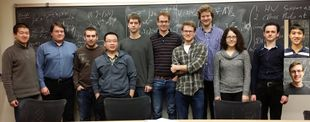© | Dror Bar-Natan: Classes: 2013-14: AKT: < >

# AKT-140404 Video

 width: 400 720 orig/AKT-140404.MOD For now, this video can only be viewed with web browsers that support

Notes on AKT-140404:    [edit, refresh]

A Monday class: back to expansions.

# Week of... Notes and Links
: Course introduction, knots and Reidemeister moves, knot colourings.
Tricolourability without Diagrams
: The Gauss linking number combinatorially and as an integral.
: The Schroedinger equation and path integrals.
Friday Introduction (the quantum pendulum)
2 Jan 13 Homework Assignment 1.
: The Kauffman bracket and the Jones polynomial.
: Euler-Lagrange problems, Gaussian integration, volumes of spheres.
3 Jan 20 Homework Assignment 2.
: The definition of finite-type and some examples.
: The self-linking number and framings.
: Integrating a polynomial times a Gaussian.
Class Photo.
4 Jan 27 Homework Assignment 3.
: Chord diagrams and weight systems.
: Swaddling maps and framings, general configuration space integrals.
: Some analysis of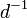$d^{-1}$.
5 Feb 3 Homework Assignment 4.
: 4T, the Fundamental Theorem and universal finite type invariants.
The Fulton-MacPherson Compactification (PDF).
: The Fulton-MacPherson Compactification, Part I.
: More on pushforwards,$d^{-1}$, and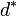$d^\ast$.
6 Feb 10 Homework Assignment 5.
: The bracket-rise theorem and the invariance principle.
: The Fulton-MacPherson Compactification, Part II.
: Gauge fixing, the beginning of Feynman diagrams.
7 Feb 24 : A review of Lie algebras.
: Graph cohomology and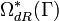$\Omega_{dR}^\ast(\Gamma)$.
: More on Feynman diagrams, beginning of gauge theory.
From Gaussian Integration to Feynman Diagrams (PDF).
8 Mar 3 Homework Assignment 6 (PDF)
: Lie algebraic weight systems.
: Graph cohomology and the construction of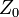$Z_0$.
Graph Cohomology and Configuration Space Integrals (PDF)
: Gauge invariance, Chern-Simons, holonomies.
Mar 9 is the last day to drop this class.
9 Mar 10 Homework Assignment 7 (PDF)
: The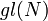$gl(N)$ weight system.
: The universal property, hidden faces.
: Insolubility of the quintic, naive expectations for CS perturbation theory.
10 Mar 17 Homework Assignment 8 (PDF)
: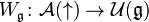$W_{\mathfrak g}\colon{\mathcal A}(\uparrow)\to{\mathcal U}({\mathfrak g})$ and PBW.
: The anomaly.
Gaussian Integration, Determinants, Feynman Diagrams (PDF).
11 Mar 24 Homework Assignment 9 (PDF)
: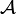${\mathcal A}$ is a bi-algebra.
: Understanding and fixing the anomaly.
Friday: class cancelled.
12 Mar 31 Monday, Wednesday: class cancelled.
: A Monday class: back to expansions.
E Apr 7 : A Friday class on what we mostly didn't have time to do.